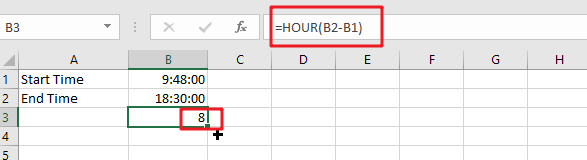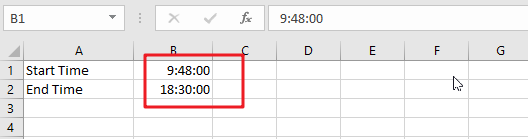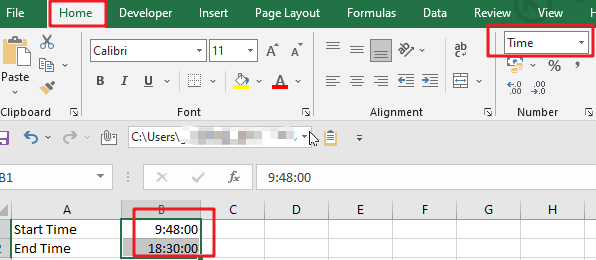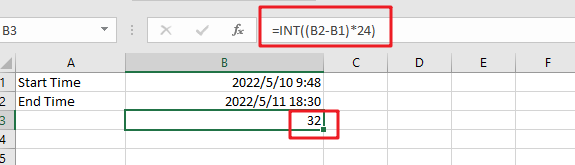# Calculate Number of Hours between Two Times

Calculating the difference between two times might be a valuable statistic for subsequent computations or averages, whether you’re producing a time sheet for staff or recording personal exercises. While Excel has a plethora of complex functions, including date and time functions, calculating time elapsed is not always obvious. If you’re calculating time differences of less than 24 hours, you may use the simple HOUR function; otherwise, you’ll need to create a new formula that accounts for longer time disparities. In this post we’ll calculate number of hours between two times.## How to use Excel to determine time difference (elapsed time)

To begin, let’s look at how to easily compute elapsed time in Excel, that is, how to determine the difference between a starting and ending time. Additionally, as is often the case, there are several formulas for doing time computations. Which one to choose is determined on the size of your dataset and the precise nature of the outcome you are attempting to attain. Therefore, let us go through each approach one by one.

## When There Are Less Than 24 Hours

Step 1: In the first cell, enter a time in the “hh:mm:ss” format and, if required, add “AM” or “PM” Enter the same information a second time in another cell using the same format. Excel will default to “AM” if you do not input “PM,” or “AM.”Step 2: Select both cells and then click the formatting window in the “Home” tab’s Number group. The formatting window’s title may read “General” or something else entirely. To format the cells as time, choose “TimeStep 3:To begin, click an empty cell. Enter an equal sign followed by the unquoted phrase “hour” Substitute an open parenthesis for the preceding. After entering the later time, click the cell with the “-” symbol. Select the first cell that corresponds to the earlier time you entered. Replace the opening parenthesis with a closing parenthesis. Subtract the first time from the second time by pressing “Enter” For instance:

`=HOUR(B2-B1)`## When 24 Hours or More

Step 1: In one cell, enter the date and time in the “mm/dd/yyy hh:mm:ss” format; in another cell, enter the date and time in the “mm/dd/yyy hh:mm:ss” format. If required, substitute “AM” or “PM” Separate the date, time, and AM or PM with spaces. For instance: 2022/5/10  9:48:00.

Step 2: Select both cells and then click the formatting window in the “Home” tab’s Number group. The formatting window’s title may read “General” or something else entirely. To open the Format Cells window, click “More Number Formats” Pick “Time” from the Category list on the left, and then navigate through the Type window’s choices to select a date and time format. Select “OK.”

Step 3: To begin, click an empty cell. Enter a “=” symbol followed by the unquoted word “INT“. Two open parentheses are required. Select the cell that contains the more recent time you entered, followed by a “” symbol. Select the first cell that corresponds to the earlier time you entered. Replace the opening parenthesis with a closing parenthesis. Without quotations, type “*24” and add the last parenthesis. “Enter.” the key. For instance:

`=INT((E8-D8)*24)`### Related Functions

• Excel HOUR Function
The Excel HOUR function returns the hour of a time value. Or returns an integer value that represent the hour component of a given time. The syntax of the HOUR function is as below:=HOUR (serial_number) …
• Excel INT function
The Excel INT function returns the integer portion of a given number. And it will rounds a given number down to the nearest integer.The syntax of the INT function is as below:= INT (number)…

Related Posts

Calculate Years Between Dates In Ms Excel

If you are an avid Ms Excel user, then you might have come across a task in which you needed to calculate the years between the dates; you might take it easy and do this task manually, which is also ...

Extract date from multiple cells containing both date and time

As if you have got a cell containing date along with the time in the same cell and you want to extract the date into a separate cell, then for one or two cells, you can extract the date manually ...

How to Auto Fill Weekdays or Weekends in Excel

Sometimes we may want to enter a sequence of days in excel for some purpose, and we can press Ctrl+; to insert current date into cell, and then drag the cell down to attach following days into other cells, then ...

How to Stack Data from Multiple Columns into One Column in Excel

In previous article, I have shown you the method to split data from one long column to multiple columns by VBA and Index function. This time if we want to stack data from multiple columns to one column, how can ...

How to Calculate Retirement Date and Remaining Years refer to Birth Date in Excel

Every company has its own police about the retirement date for employees. Suppose employees will be retired from a certain age of 60, how can we calculate the retirement date for everyone base on their birthdays? Actually, there are several ...

How to List Only Whole Numbers From Decimal Numbers in Excel

This post will guide you how to filter only whole numbers from decimal numbers in a list in Excel. How do I filter cells with whole numbers or non-whole numbers in Excel. List Only Whole Numbers Assuming that you have ...

How to Calculate Number of Weekends between Two Dates in Excel

This post will guide you how to count the number of weekends between two given dates in Excel. How to find out how many weekends are between two dates in Excel. How to count the number of Sundays between two ...

How to Check If a Number is Integer in Excel

This post will guide you how to check if a number is integer in Cells in Excel. How do I test if Cell value is integer with a formula in Excel. Check If Value is Integer Assuming that you have ...

How to Delete Numbers after Decimal Point in Excel

This post will guide you how to remove decimals without rounding in Excel. How do I delete numbers after decimal point with a formula in the given cell in Excel. Deleting Numbers after Decimal Point Assuming that you have a ...

Count the Number of Weekends between Two Date

This post will guide you how to count the number of weekends between two dates in Excel. How do I get the number of weekends between 2 dates with a formula in Excel. How to count weekend days between dates ...

Sidebar